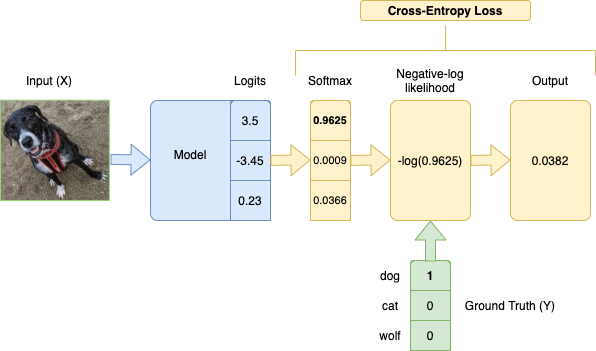## Categorical Cross-Entropy Loss

Categorical Cross-Entropy Loss Function, also known as Softmax Loss, is a loss function used in multiclass classification model training. It applies the Softmax Activation Function to a model's output (logits) before applying the Negative Log-Likelihood function.

Lower loss means closer to the ground truth.

In math, expressed as

$P = \text{softmax}(O)$

$-\sum\limits_{i=1}^{N} Y_{i} \times \log(P_{i})$

where $N$ is the number of classes, $Y$ is the ground truth labels, and $O$ is the model outputs. Since $Y$ is one-hot encoded, the labels that don't correspond to the ground truth will be multiplied by 0, so we effectively take the log of only the prediction for the true label.Based on Cross-Entropy in Information Theory, which is a measure of difference between 2 probability distributions.

Howard et al. (2020) (pg. 222-231)

#### References

Jeremy Howard, Sylvain Gugger, and Soumith Chintala. Deep Learning for Coders with Fastai and PyTorch: AI Applications without a PhD. O'Reilly Media, Inc., Sebastopol, California, 2020. ISBN 978-1-4920-4552-6.